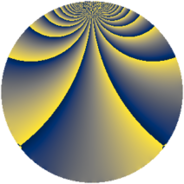Properties

 Label 1050.3.bmLevel $1050$ Weight $3$ Character orbit 1050.bm Rep. character $\chi_{1050}(11,\cdot)$ Character field $\Q(\zeta_{30})$ Dimension $1280$ Sturm bound $720$

Related objects

Defining parameters

 Level: $$N$$ $$=$$ $$1050 = 2 \cdot 3 \cdot 5^{2} \cdot 7$$ Weight: $$k$$ $$=$$ $$3$$ Character orbit: $$[\chi]$$ $$=$$ 1050.bm (of order $$30$$ and degree $$8$$) Character conductor: $$\operatorname{cond}(\chi)$$ $$=$$ $$525$$ Character field: $$\Q(\zeta_{30})$$ Sturm bound: $$720$$

Dimensions

The following table gives the dimensions of various subspaces of $$M_{3}(1050, [\chi])$$.

Total New Old
Modular forms 3904 1280 2624
Cusp forms 3776 1280 2496
Eisenstein series 128 0 128

Trace form

 $$1280 q - 320 q^{4} + 8 q^{7} + O(q^{10})$$ $$1280 q - 320 q^{4} + 8 q^{7} + 8 q^{10} - 52 q^{15} + 640 q^{16} + 32 q^{18} - 60 q^{21} + 112 q^{22} - 20 q^{25} + 456 q^{27} + 24 q^{28} - 56 q^{30} - 60 q^{31} + 30 q^{33} + 64 q^{37} - 20 q^{39} - 16 q^{40} + 160 q^{42} - 64 q^{43} + 334 q^{45} + 120 q^{46} + 120 q^{49} - 120 q^{51} + 392 q^{55} + 1368 q^{57} + 112 q^{58} + 28 q^{60} + 322 q^{63} + 2560 q^{64} - 160 q^{66} - 32 q^{67} + 420 q^{69} + 1008 q^{70} - 64 q^{72} - 336 q^{73} - 970 q^{75} - 256 q^{78} + 280 q^{79} + 40 q^{81} + 512 q^{82} - 180 q^{84} + 1880 q^{85} + 652 q^{87} - 128 q^{88} - 128 q^{90} - 340 q^{91} + 244 q^{93} + 160 q^{94} + 648 q^{97} + 320 q^{99} + O(q^{100})$$

Decomposition of $$S_{3}^{\mathrm{new}}(1050, [\chi])$$ into newform subspaces

The newforms in this space have not yet been added to the LMFDB.

Decomposition of $$S_{3}^{\mathrm{old}}(1050, [\chi])$$ into lower level spaces

$$S_{3}^{\mathrm{old}}(1050, [\chi]) \cong$$ $$S_{3}^{\mathrm{new}}(525, [\chi])$$$$^{\oplus 2}$$